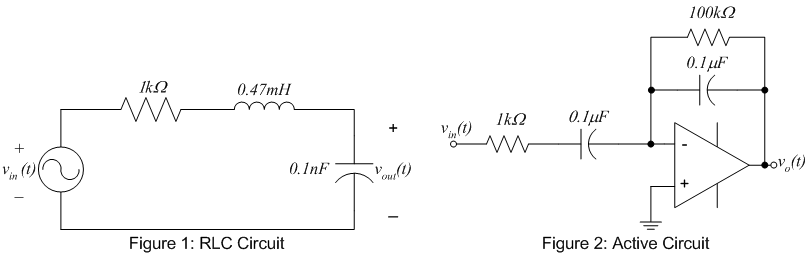EE 212 Lab
Lab 6: Frequency Response of Circuits

The purpose of this lab is to experimentally construct the frequency responses of two circuits. The circuits to be studied are the passive RLC circuit shown in figure 1 and the active network shown in figure 2.1. Frequency response of RLC circuit.

• Construct the circuit shown in figure 1.
• Let vin(t) be a 1 Vp-p sine wave for which you'll vary the frequency.
• Use your prelab results as a guide as to what frequency range should be used and measure the frequency response (magnitude and phase) of Vout(jw)/Vin (jw). Note the function generator and oscilloscope display frequency in Hertz and your Bode plots are likely versus frequency in rad/sec, so make sure to convert whichever way you choose to keep the units consistent. Take extra points in regions where the magnitude or phase change quickly.
• Compare your results with the calculated values plotted in the prelab by recording the experimental data on top of the calculated prelab data.
• How would one describe this circuit?
• Does this passive circuit ever yield a gain larger than 1 (0dB)? If so, how is this possible?

2. Frequency response of active circuit.

• Construct the circuit shown in figure 2.  Use your LM741 Operational Amplifier. The pinout for
this device can be found in the LM741 spec sheet.  Do not print out the entire spec. sheet!
• Let vin(t) be a 1 Vp-p sine wave for which you'll vary the frequency.
• Use your prelab results as a guide to what frequency range should be used and measure the frequency response (magnitude and phase) of Vout(jw)/ Vin (jw). Note the function generator and oscilloscope display frequency in Hertz and your Bode plot may display frequency in rad/sec, so make sure to convert whichever way you choose to keep the units consistent. Take extra points in regions where the magnitude or phase change quickly.
• Compare your results with the calculated values plotted in the prelab by recording the experimental data on top of the calculated prelab data.
• How would one describe this circuit?